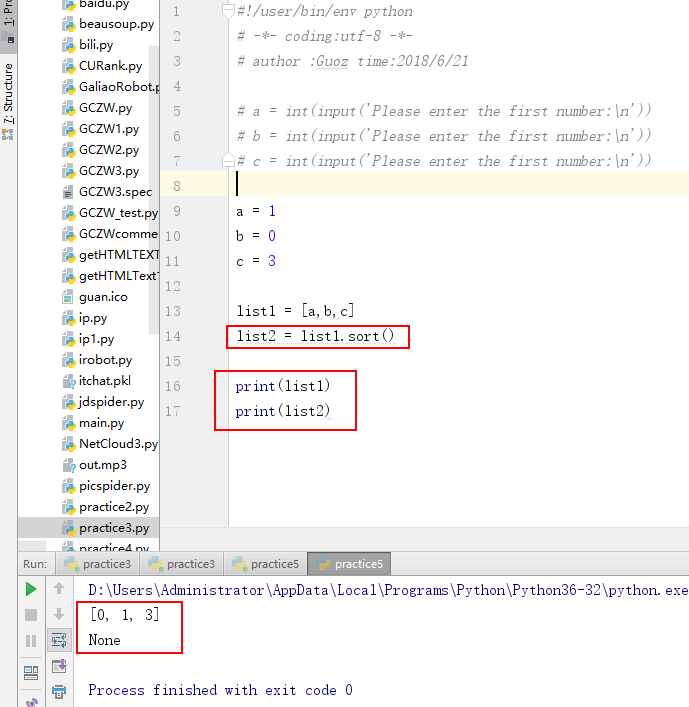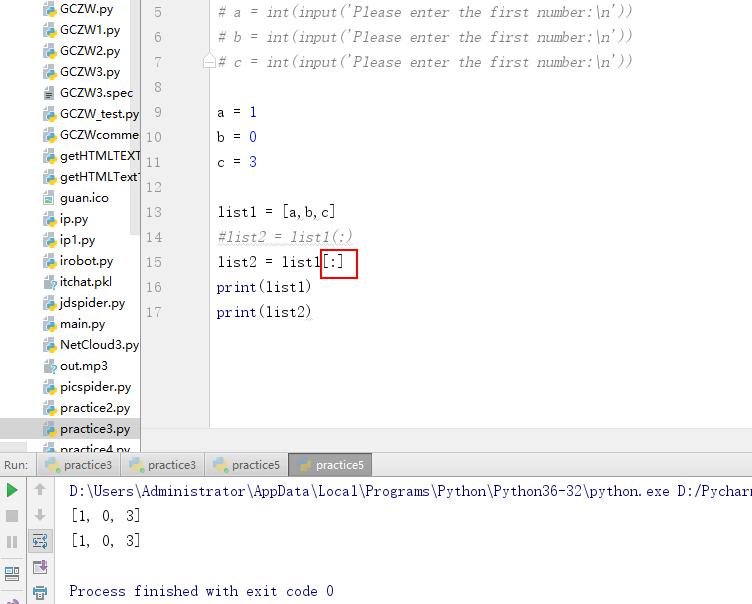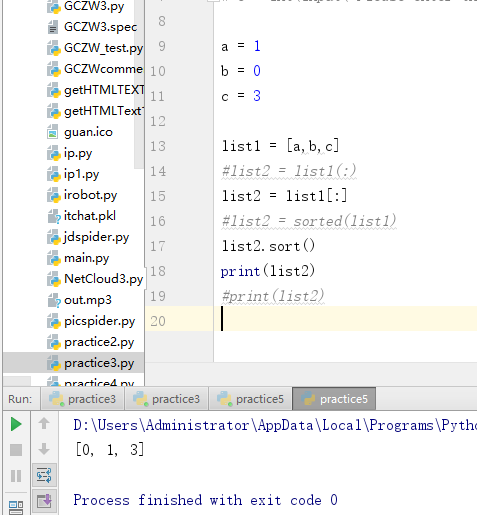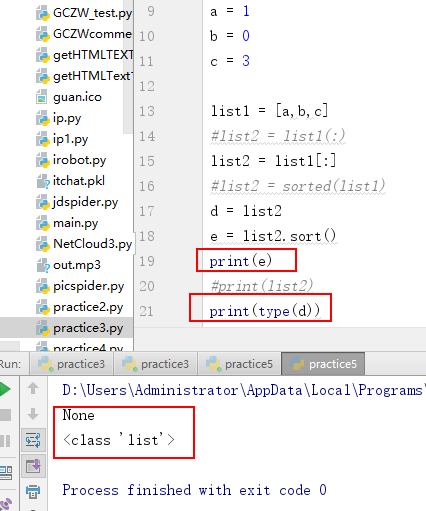# Python100例实训（一）

##### 古人之观于天地、山川、草木、虫鱼、鸟兽，往往有得，以其求思之深而无不在也。夫夷以近，则游者众；险以远，则至者少。而世之奇伟、瑰怪，非常之观，常在于险远，而人之所罕至焉，故非有志者不能至也。有志矣，不随以止也，然力不足者，亦不能至也。有志与力，而又不随以怠，至于幽暗昏惑而无物以相之，亦不能至也。然力足以至焉，于人为可讥，而在己为有悔；尽吾志也而不能至者，可以无悔矣，其孰能讥之乎？

Practice makes perfect

#### Python 实例1

for i in range(1,5):
for j in range(1,5):
for k in range(1,5):
if( i != k ) and (i != j) and (j != k):
print i,j,k

#### Python 实例2

#p = 120000
p = int(input('请输入利润：'))
profit = [1000000,600000,400000,200000,100000,0]
ratio = [0.01,0.015,0.03,0.05,0.075,0.1]
r = 0
for idx in range(0,6):
if p>profit[idx]:
r += (p - profit[idx])*ratio[idx]
print ((p - profit[idx])*ratio[idx])
p = profit[idx]
print(r)

Python 实例3

import math

x = range(0,1000000)
for i in x:
y = i+100
if math.sqrt(y)%2 == 1 or math.sqrt(y)%2 == 0:
z = y + 168
if math.sqrt(z)%2 == 1 or math.sqrt(z)%2 == 0:
print(i,y,z)

Python 实例4

#### 输入某年某月某日，判断这一天是这一年的第几天？

dayth = 0
inputyear = int(input('Please enter the YEAR:\n'))
inputmonth = int(input('Please enter the MONTH:\n'))
inputday = int(input('Please enter the DAY:\n'))

daysOFmonthP = {1: 31, 2: 28, 3: 31, 4: 30, 5: 31, 6: 30, 7: 31, 8: 31, 9: 30, 10: 31, 11: 30, 12: 31}
daysOFmonthR = {1: 31, 2: 29, 3: 31, 4: 30, 5: 31, 6: 30, 7: 31, 8: 31, 9: 30, 10: 31, 11: 30, 12: 31}

if (inputyear % 4 == 0 or inputyear % 400 == 0) and inputyear % 100 != 0:
alldays = 366
monthDaydict = daysOFmonthR
else:
alldays = 365
monthDaydict = daysOFmonthP

for i in range(1, inputmonth):
dayth = dayth + monthDaydict[i]

print('The day is %dth day in the year' % (dayth+inputday))#### 第一种：用sorted函数

a = int(input('Please enter the first number:\n'))
b = int(input('Please enter the first number:\n'))
c = int(input('Please enter the first number:\n'))
list1 = [a,b,c]
list2 = sorted(list1)
print(list2)

a = int(input('Please enter the first number:\n'))
b = int(input('Please enter the first number:\n'))
c = int(input('Please enter the first number:\n'))

list1 = [a,b,c]
list2 = list1[:]

d = list2
e = list2.sort()
#print(type(d))
print(e)y = x[:] 通过分片操作将列表x的元素全部拷贝给y，如果简单的把x赋值给y：y = x，y和x还是指向同一个列表，并没有产生新的副本。#p = 120000

python3中list用了sort()方法后是None09-19453

#### Python100例实训（二）06-02212

#### Python爬虫综合实验

01-053768

#### python实例100例精选50例（基于python3.7，数据分析方向）

01-229858

#### Python数据分析与应用课后实训（一）

10-254220

#### python实践项目(一)©️2020 CSDN 皮肤主题: 大白 设计师: CSDN官方博客点击重新获取扫码支付1.余额是钱包充值的虚拟货币，按照1:1的比例进行支付金额的抵扣。
2.余额无法直接购买下载，可以购买VIP、C币套餐、付费专栏及课程。余额充值